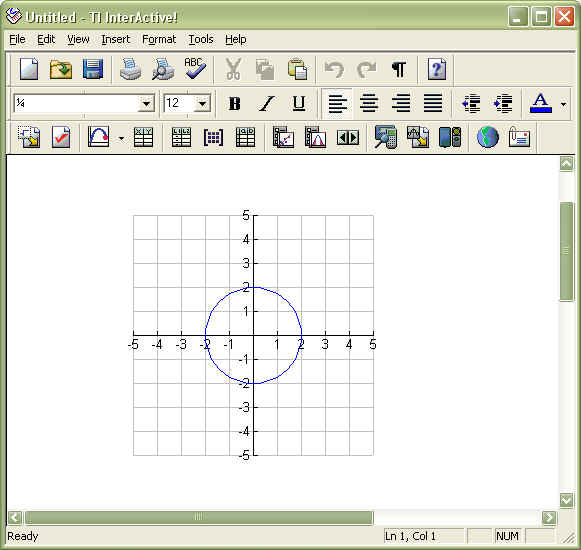# Knowledge Base

## Solution 13365: Graphing Parametric Functions with TI Interactive!™ Software.

### How can I graph parametric functions with TI Interactive! software?

The example below shows how to graph a parametric function with TI Interactive! software:

Example: Graph x(t)=2cos(t) and y(t)=2sin(t)

Solution:

• Open a new TI Interactive document
• Click Insert on the TI Interactive menu bar, and select Graph> Parametric
• In the text boxes, enter the parametric equationsx1(t)=2cos(t) and y1(t)=2sin(t) and click Close
• Adjust the graph to zoom in on the circles. Click Zoom from the menu bar and Set Xmin=-5,Xmax=5, Ymin=-5, and Ymax=5
• Click the Save to Document icon to paste the graph into the document